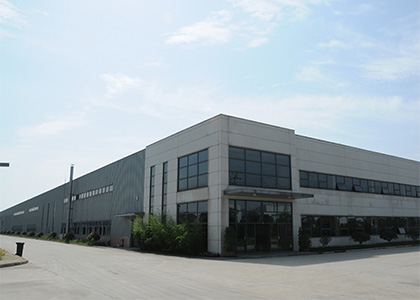Product center
•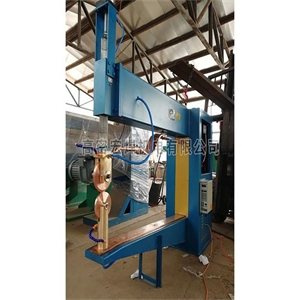水塔设备
•水塔设备
•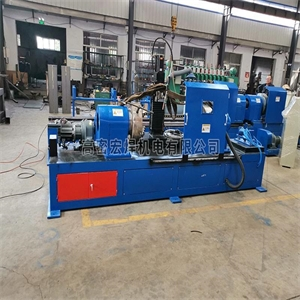自动绕网焊机
•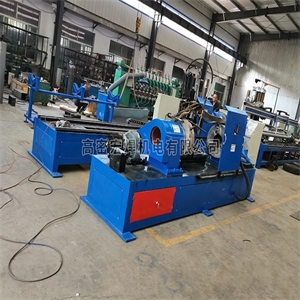自动切管机
•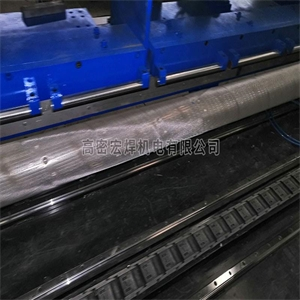自动滤网点焊机
•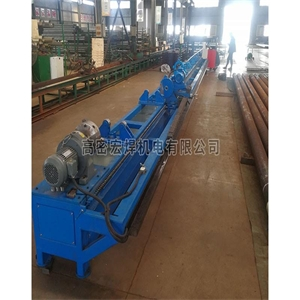自动焊机工装
•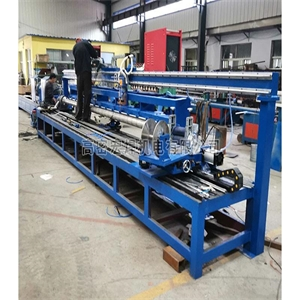自动点焊机
•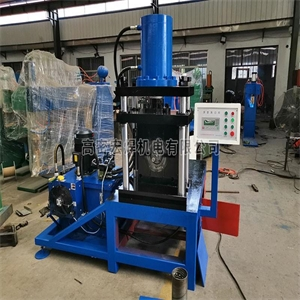胀管机
•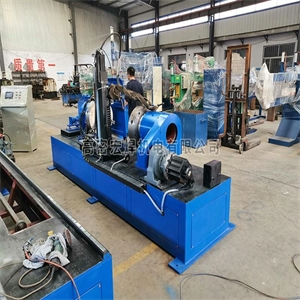数控自动焊接机
•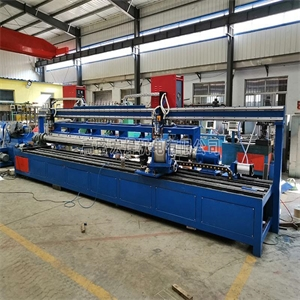数控自动焊机
•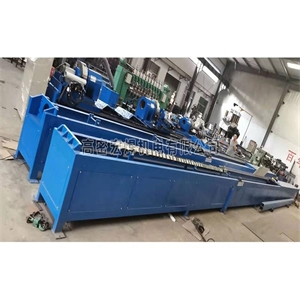数控工装
•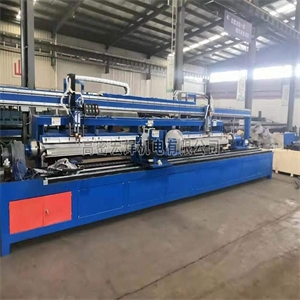石油筛管自动焊机
•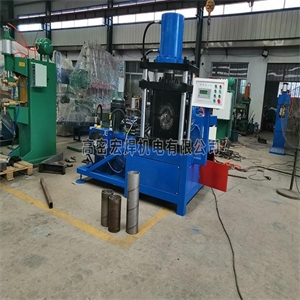石油筛管收口机
•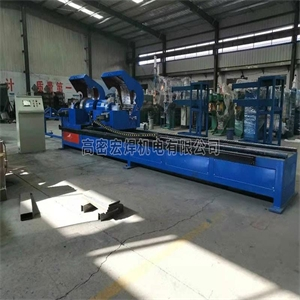石油筛管切断机
•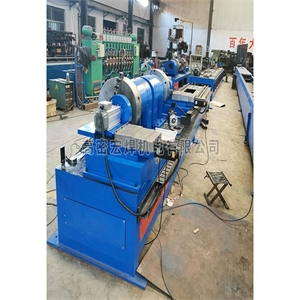石油筛管焊机
•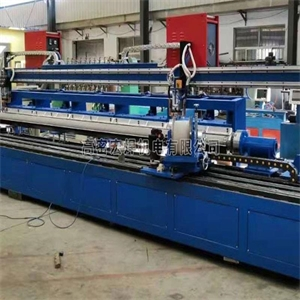石油筛管点焊机
•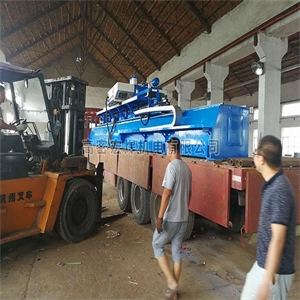石油机械自动焊机
•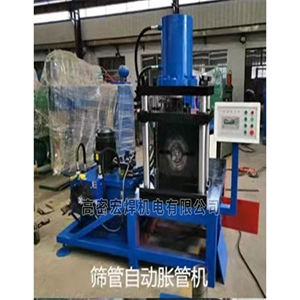筛管自动涨管机
•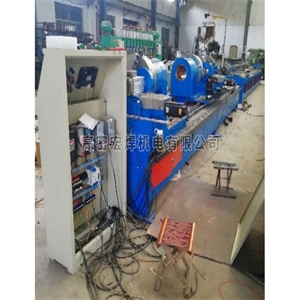筛管自动切割锯
•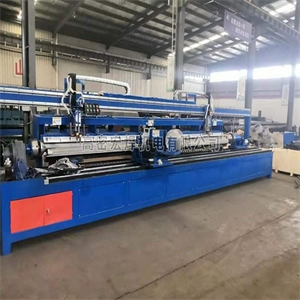筛管自动焊
•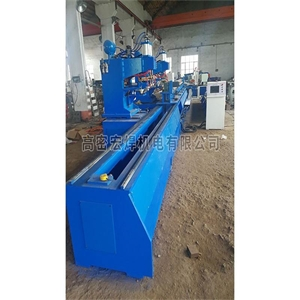筛管自动点焊机
•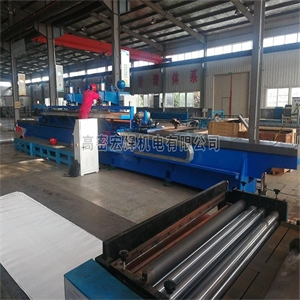筛管缠绕网机
•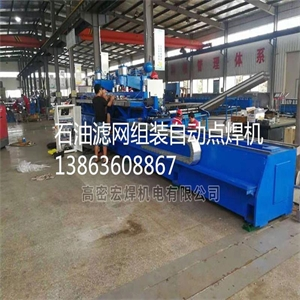滤网组装点焊机
•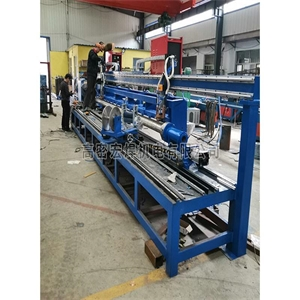滤网点焊机
•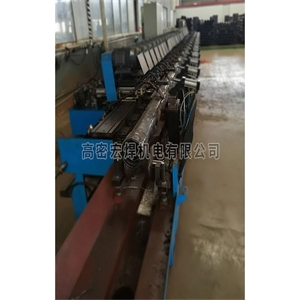螺旋管焊机
•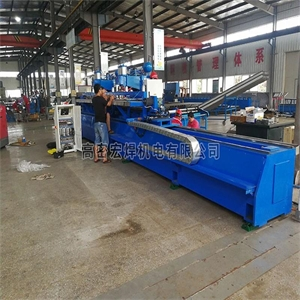机械自动焊
•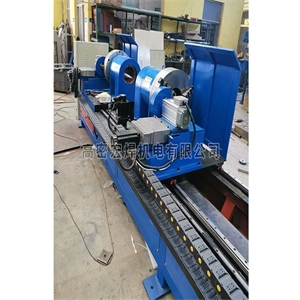管材定尺切断机
•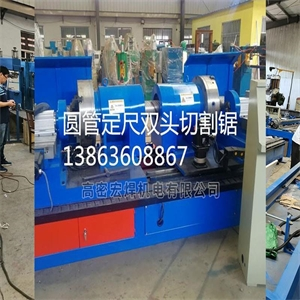定尺自动切割锯
•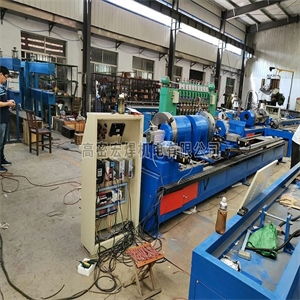石油筛管设备
•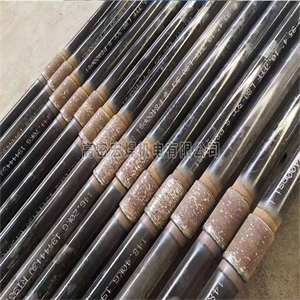石油筛管设备
•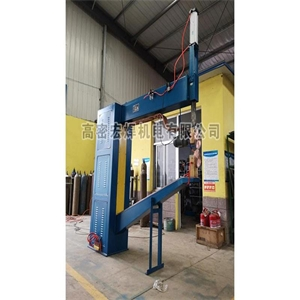直流缝焊机
•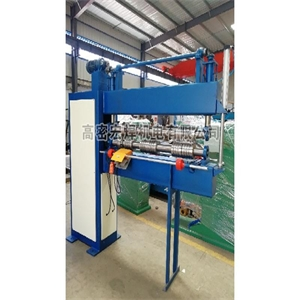压筋机
•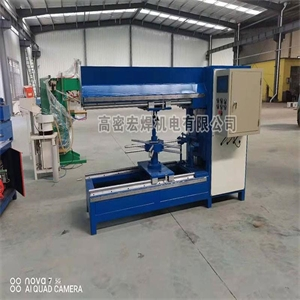水塔盖子翻边机
•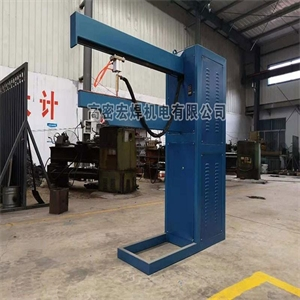轨道点焊机
•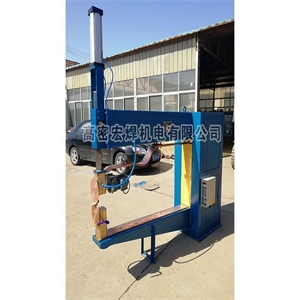二用缝焊机
•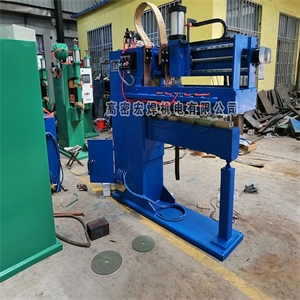自动直缝焊机
•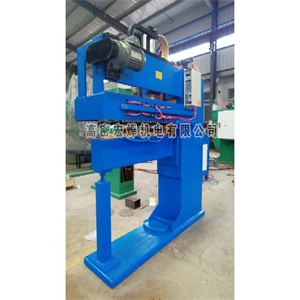自动压紧滚焊机
•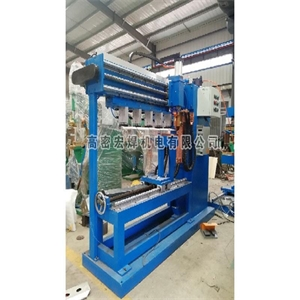自动焊缝机
•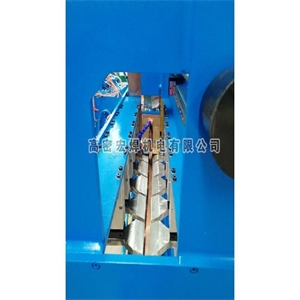自动缝焊机
•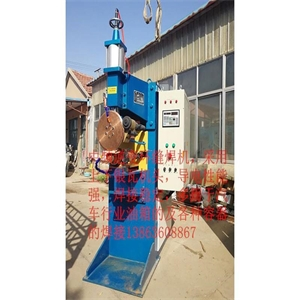中频逆变环缝焊机
•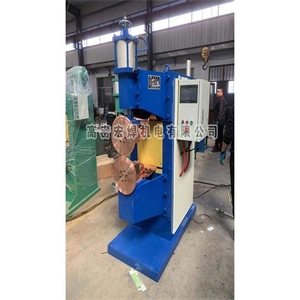中频缝焊机
•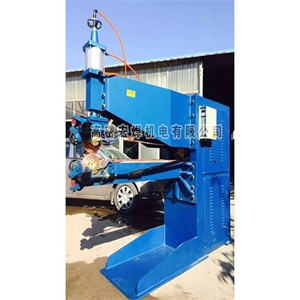直缝焊机
•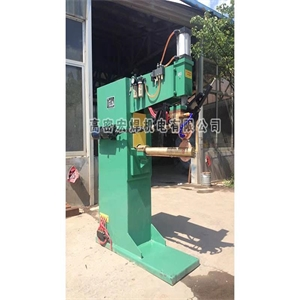直缝焊机
•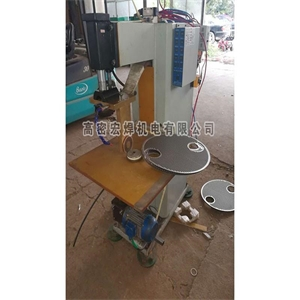圆角焊机
•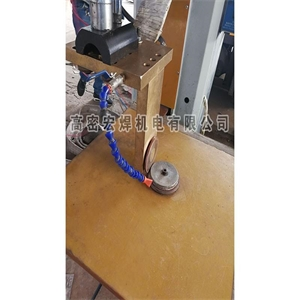圆角缝焊机
•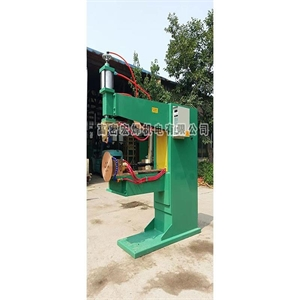银瓦机头缝焊机
•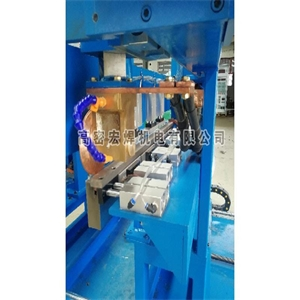箱体缝焊机
•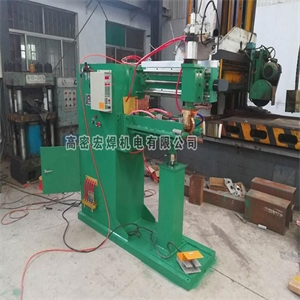丝网焊机
•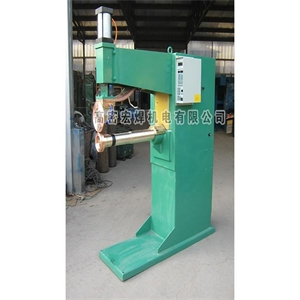手提油桶环缝焊机
•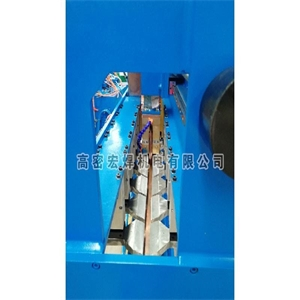上动式直缝焊机
•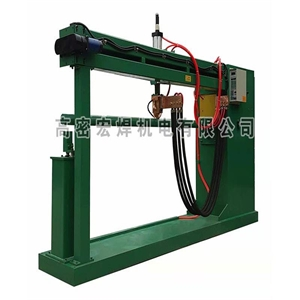上动缝焊机
•配电箱专用缝焊机
•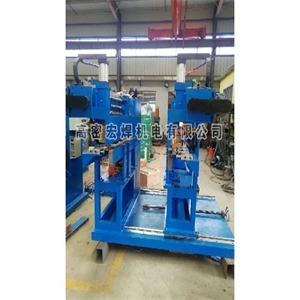配电箱底焊缝机
•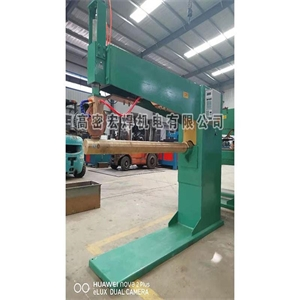加长缝焊机
•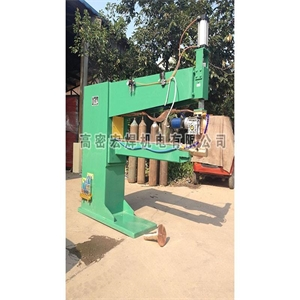环直二用缝焊机
•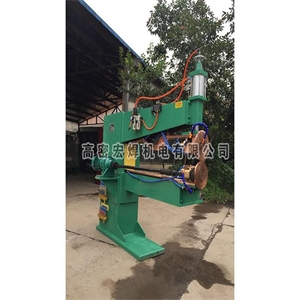环缝焊机
•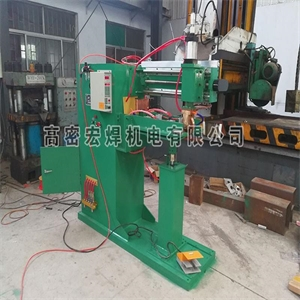过滤网自动直缝焊机
•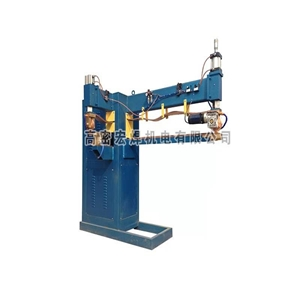工程联箱焊机
•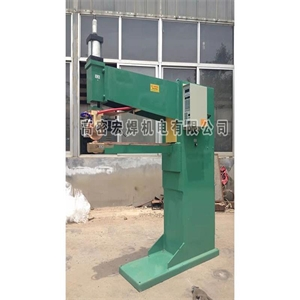缝焊机
•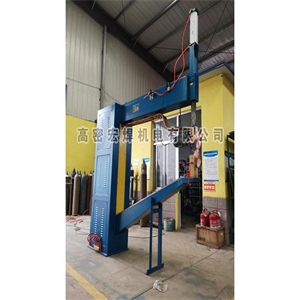大型水箱缝焊机
•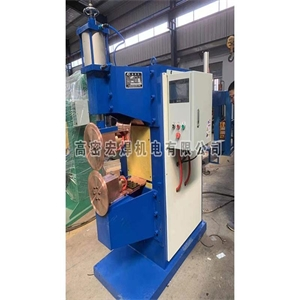变频缝焊机
•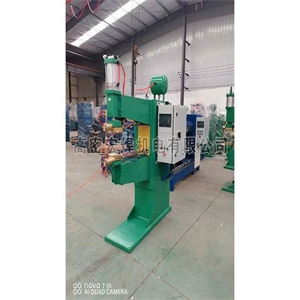中频逆变点焊机
•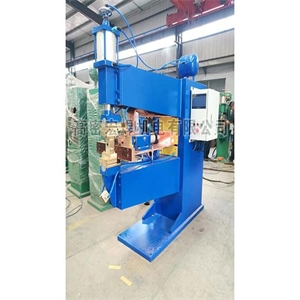中频多功能点焊机
•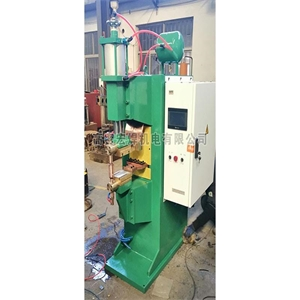中频点焊机
•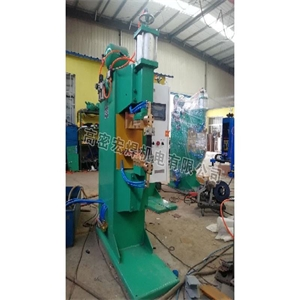中频点焊机-(2)
•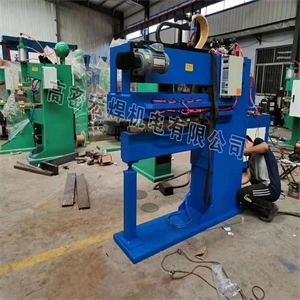轧钢带焊机
•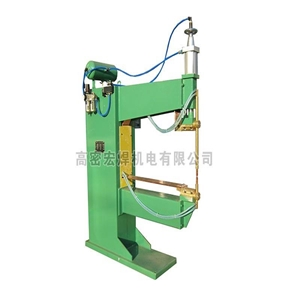水槽台面焊机加长
•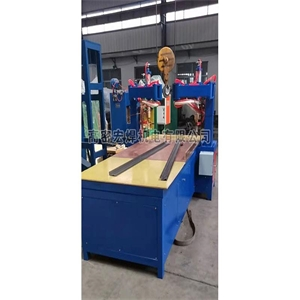双头摇臂点焊机
•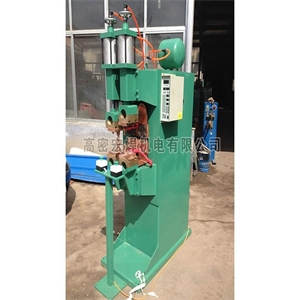双头螺母点焊机
•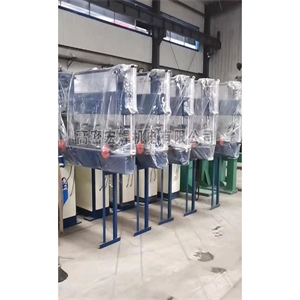双电动滚筋机
•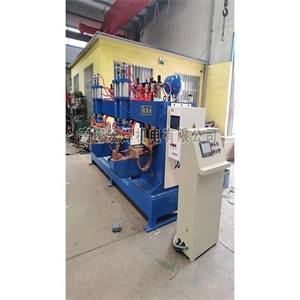数控自动焊机
•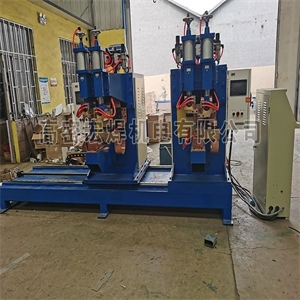数控自动点焊机
•数控中频点焊机
•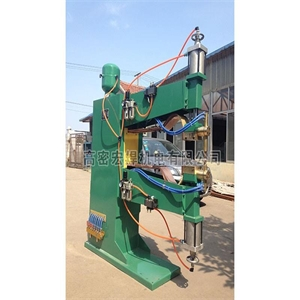上下气动双动点焊机
•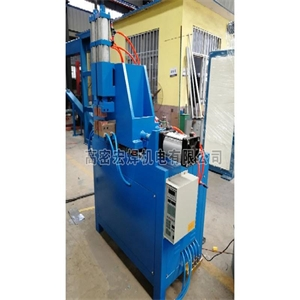气动对焊机
•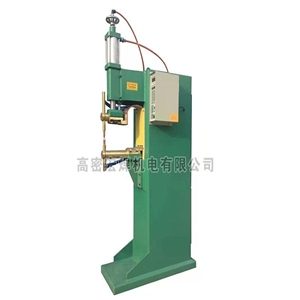气动点焊机-(2)
•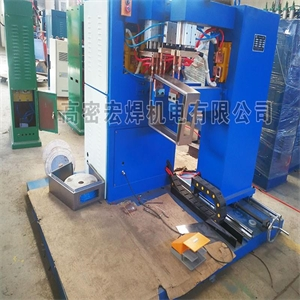配电箱自动焊机
•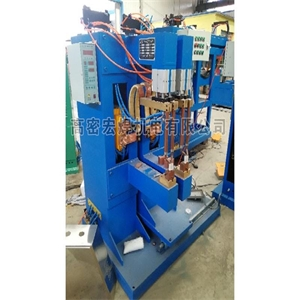配电箱焊接专机
•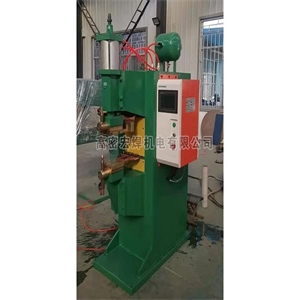逆变点焊机
•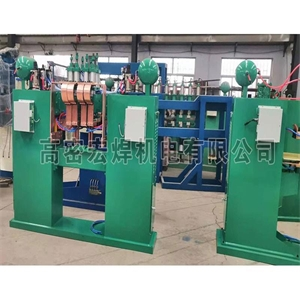龙门排焊机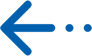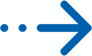•厂家直销

•经验丰富

•精益求精

•产品实拍

•真诚服务

•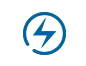闪电发货

•贴心包装

•及时物流